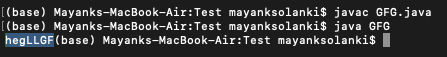# Java Program to Move All Uppercase Characters to the End

• Last Updated : 09 Jun, 2021

Given a string that contains uppercase letters as well as lower case letters. The task is to move all uppercase characters at the end of the String. The uppercase characters must be in the same order as in the original string.

```Input    : "heLLGFg"
Output   : "hegLLGF"

Input    : "Hello"
Output   : "elloH"```

Here we are having two different approaches to get to the problem namely as follows:

Attention reader! Don’t stop learning now. Get hold of all the important Java Foundation and Collections concepts with the Fundamentals of Java and Java Collections Course at a student-friendly price and become industry ready. To complete your preparation from learning a language to DS Algo and many more,  please refer Complete Interview Preparation Course.

1. Using ASCII values of the characters.
2. Using queue data structures

Approach 1: Using ASCII values of the characters.

• Iterate the character of the string one by one and checks the ASCII values of the respective character of the string.
• For all uppercase letters, ASCII values lie under [65-90] char values. Store the characters of these ASCII values in the String variable and print the string at the end of Iteration.

Example:

## Java

 `// Java Program to Move All Uppercase Characters to the End` `// Importing input output classes``import` `java.io.*;` `// Mai class``class` `GFG {` `    ``// Method 1``    ``// To shift uppercase characters``    ``static` `void` `shiftuppercase(String m, ``int` `length)``    ``{` `        ``// Taking an empty string``        ``String temp = ``""``;` `        ``for` `(``int` `i = ``0``; i < length; ++i) {` `            ``// Condition check``            ``// If the character is uppercase via``            ``// the ASCII values of the  character``            ``if` `(m.charAt(i) >= ``65` `&& m.charAt(i) <= ``90``) {``                ``temp += m.charAt(i);``            ``}` `            ``// The character is already lowercase``            ``else``                ``System.out.print(m.charAt(i));``        ``}` `        ``// Now, Printing the uppercase string``        ``System.out.print(temp);``    ``}` `    ``// Method 2``    ``// Main driver method``    ``public` `static` `void` `main(String[] args)``    ``{``        ``// Custom input string``        ``String m = ``"heLLGFg"``;` `        ``// Computing the length of the string``        ``// using length() method``        ``int` `length = m.length();` `        ``// Calling the method 1 over the custom string taken``        ``// above to move all uppercase char to the end``        ``shiftuppercase(m, length);``    ``}``}`

Output:Approach 2: Using queue data structures

• Queue Data structure implements First in First out concept, We use this concept and store the uppercase characters in the queue.
• Print all the characters except the uppercase characters.
• After the iteration removes all the elements from the queue.

Example:

## Java

 `// Java Program to Move All Uppercase Characters to the End``// Using Queue data structures` `// Importing input output classes``import` `java.io.*;``// Importing utility classes``import` `java.util.*;` `// Main class``class` `GFG {` `    ``// Method 1``    ``// Main driver method``    ``static` `void` `shiftuppercase(String m, ``int` `length)``    ``{``        ``// Creating an object of Queue class of character``        ``// type``        ``Queue Q = ``new` `LinkedList();` `        ``// Declaring an initializing to empty string``        ``String temp = ``""``;` `        ``for` `(``int` `i = ``0``; i < length; ++i) {` `            ``// Condition checkfor the uppercase characters``            ``// If uppercase use ASCII values of the``            ``// character``            ``if` `(m.charAt(i) >= ``65` `&& m.charAt(i) <= ``90``) {``                ``Q.add(m.charAt(i));``            ``}` `            ``// Character is lowercase``            ``else` `                ``// Leave it asities on its index``                ``System.out.print(m.charAt(i));``        ``}` `        ``// Now, printing the uppercase string till``        ``// there are elements in queue``        ``while` `(Q.size() != ``0``) {` `            ``// Removing all the elements from the queue``            ``System.out.print(Q.peek());` `            ``// Clear the queue``            ``Q.remove();``        ``}``    ``}` `    ``// Method 2``    ``// main driver method``    ``public` `static` `void` `main(String[] args)``    ``{` `        ``// Given input string``        ``String m = ``"heLLGFg"``;` `        ``//  Computing the length of the string``        ``// using length() method``        ``int` `length = m.length();` `        ``// Calling the``        ``shiftuppercase(m, length);``    ``}``}`

Output:My Personal Notes arrow_drop_up